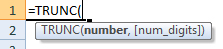# TRUNC Function – Truncate in Excel, VBA, & Google Sheets

This tutorial demonstrates how to use the Excel TRUNC Function in Excel to truncate a number.

## TRUNC Function Overview

The TRUNC Function Truncates a number to a specific number of digits.

To use the TRUNC Excel Worksheet Function, select a cell and type:(Notice how the formula inputs appear)

### TRUNC Function Syntax and Inputs:

`=TRUNC(number,num_digits)`

number – A number.

num_digits – The number of decimal places you want remaining after you truncate. Zero for none. Negative will truncate to the left of the decimal place.

## TRUNC Examples in VBA

TRUNC is not a member of the application.worksheetfunction. Because of this, it cannot be used the same way from within Excel VBA. Instead, we can use the FORMAT function, which will create the same result, as follows:

Executing the following VBA statements

For the function arguments (number, etc.), you can either enter them directly into the function, or define variables to use instead.